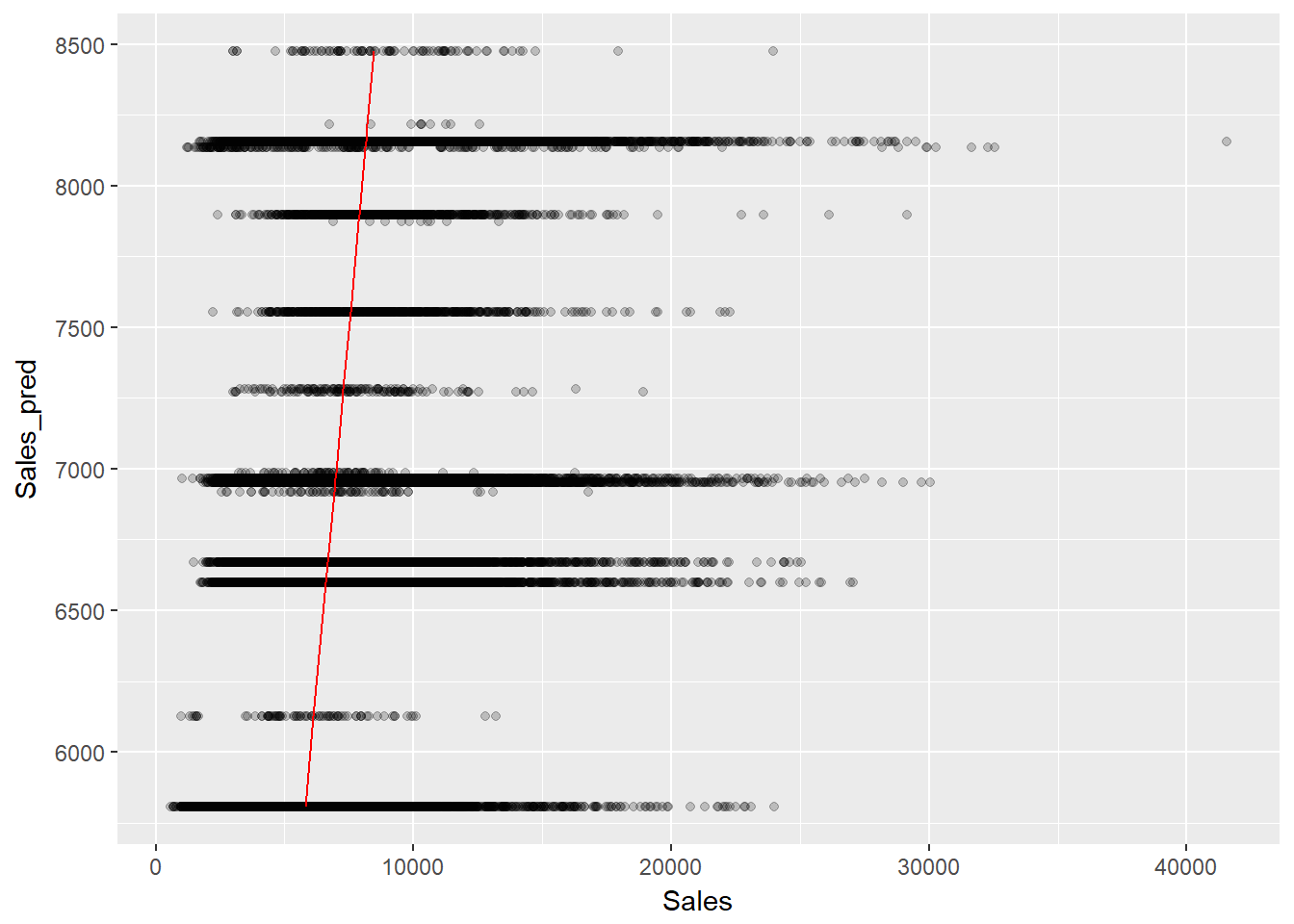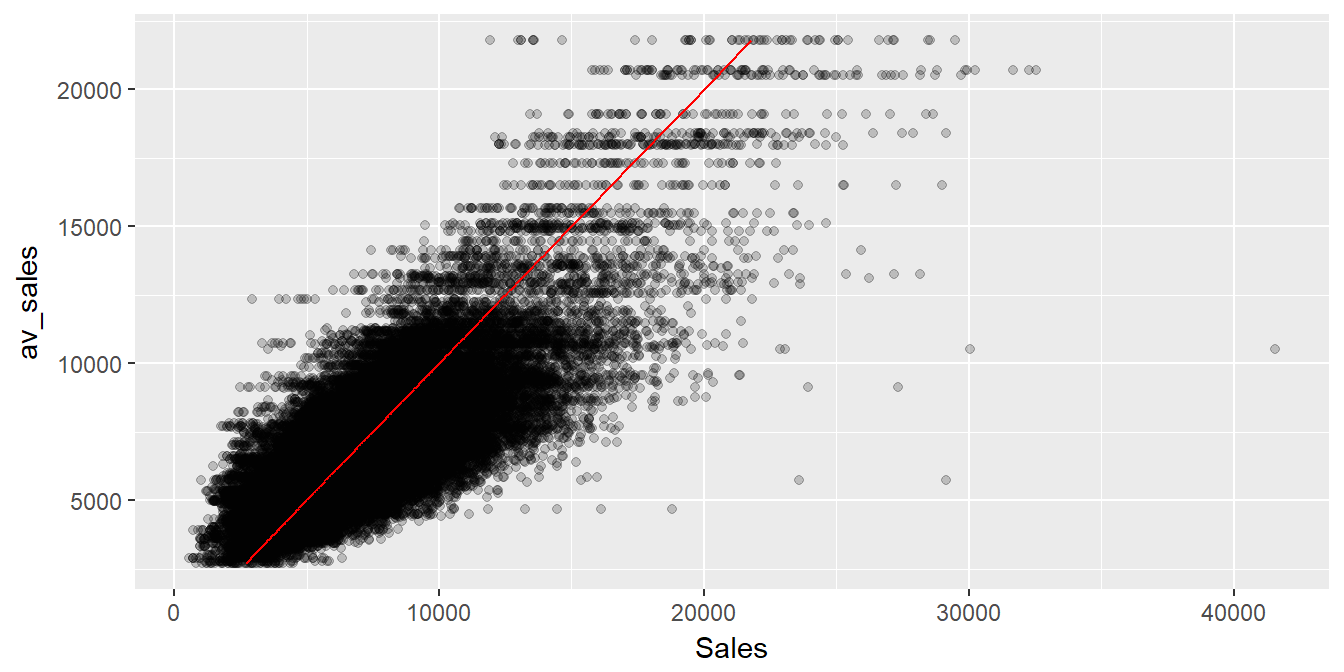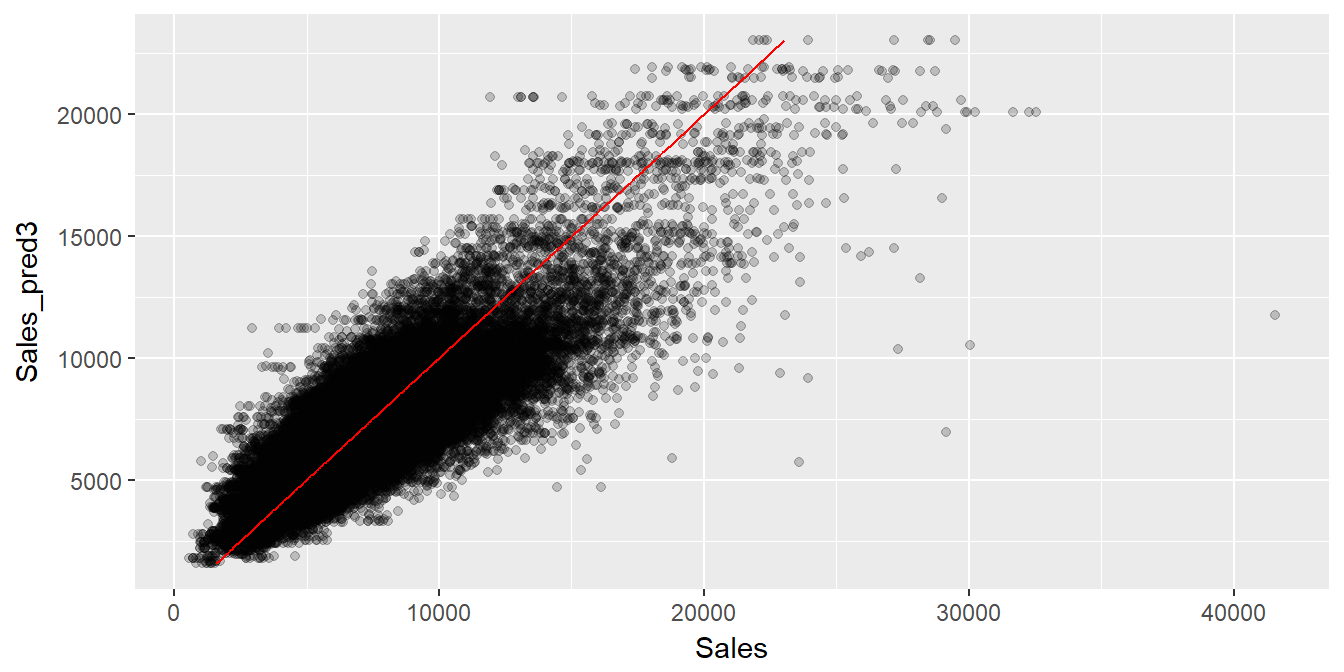# Lab 15: Regression Analysis II

### Learning objectives:

• estimate a regression
• predict using a linear regression model
• evaluate predictions using Mean Square Error and its variants

### 0. Load the data

Let’s load and slightly massage the Rossmann data.

library(tidyverse) #includes dplyr, ggplot, tidyr, readr
library(lubridate)
library(stargazer)
#load in sales and fix the date
sales <- read_csv("../rossmann_train.csv", col_types = cols(StateHoliday = col_character()))
sales$day <- wday(sales$Date, label = TRUE, abbr = TRUE) #creates 'ordered factor'
sales$day <- factor(sales$day, ordered=FALSE) # makes it a factor
sales <- select(sales, -DayOfWeek) #not needed anymore

#load in stores info and create competition date (compdate) variable
stores <- read_csv("../rossmann_store.csv")
stores$compdate <- as.Date(paste(stores$CompetitionOpenSinceYear,stores$CompetitionOpenSinceMonth,15, sep="-")) stores$compdate[is.na(stores$CompetitionOpenSinceYear)] <- as.Date("2000-01-01") #merge store info into sales data sales <- inner_join(sales, select(stores, Store, compdate), by="Store") #create competition yes or no variable sales$competition <- ifelse(sales$Date<sales$compdate, "no", "yes")
# create before and after holidays variable
sales <- arrange(sales, Store, Date)
sales$before <- ifelse(lead(sales$StateHoliday)!="0" & sales$Store==lead(sales$Store),
"yes", "no")
#assume the last day in the dataset is NOT before holiday
sales$before <- ifelse(is.na(sales$before), "no", sales$before) sales$after  <- ifelse(lag(sales$StateHoliday)!="0" & sales$Store==lag(sales$Store), "yes","no") #assume the first day in the dataset is NOT after holiday sales$after <- ifelse(is.na(sales$after), "no", sales$after)

#drop days when stores are closed
sales <- sales %>% filter(Open==1) %>% select(-Open)

#let's save this massaged data for future use
write_csv(sales, "rossmann_massaged.csv")

### 1. Estimate a regression

Now that we are familiar with the Rossmann data set, let’s use regression analysis to try to predict store sales. Let’s spit the data into test and train data sets. As test data set let’s use data through May 31st, 2015. As test data, we will use data from June 1st to July 31st, 2015.

train <- filter(sales, Date < as.Date("2015-06-01"))
test <-  filter(sales, Date >= as.Date("2015-06-01"))

The regression function is lm(). It takes a formula as its first argument and data as the second argument. Let’s use Sales as our dependent. Let’s start with day as our explanatory variable.

reg1 <- lm(Sales ~ day , data=train)
summary(reg1)
##
## Call:
## lm(formula = Sales ~ day, data = train)
##
## Residuals:
##    Min     1Q Median     3Q    Max
##  -8191  -2045   -534   1388  31655
##
## Coefficients:
##             Estimate Std. Error t value Pr(>|t|)
## (Intercept)  8208.79      52.41 156.640   <2e-16 ***
## dayMon        -17.84      53.09  -0.336    0.737
## dayTue      -1153.11      53.05 -21.735   <2e-16 ***
## dayWed      -1503.06      53.06 -28.326   <2e-16 ***
## dayThu      -1445.64      53.10 -27.226   <2e-16 ***
## dayFri      -1142.20      53.08 -21.518   <2e-16 ***
## daySat      -2321.77      53.05 -43.767   <2e-16 ***
## ---
## Signif. codes:  0 '***' 0.001 '**' 0.01 '*' 0.05 '.' 0.1 ' ' 1
##
## Residual standard error: 3026 on 785774 degrees of freedom
## Multiple R-squared:  0.04887,    Adjusted R-squared:  0.04887
## F-statistic:  6729 on 6 and 785774 DF,  p-value: < 2.2e-16

day is a qualitative variable which can take any one of seven possible values. Notice that R understood that it needed to create a set of six dummy variables to estimate the effect of different days of the week. It left out the first factor level, Sunday. Can you interpret the coefficient on Saturday?

Let’s add our competiton and then our before and after variables to the right-hand side. We will present results from all three regressions using stargazer. When we feed stargazer regression objects, it understands to present the results in standard form (each regression in a separate column.)

reg2 <- lm(Sales ~ day + competition, data=train)
reg3 <- lm(Sales ~ day + competition + before + after, data=train)

stargazer(reg1, reg2, reg3, type="text", digits = 2, keep.stat=c("rsq","adj.rsq","n"))
##
## =====================================================
##                         Dependent variable:
##                --------------------------------------
##                                Sales
##                    (1)          (2)          (3)
## -----------------------------------------------------
## dayMon            -17.84       -29.75       20.82
##                  (53.09)      (53.06)      (52.93)
##
## dayTue         -1,153.11*** -1,164.90*** -1,182.04***
##                  (53.05)      (53.03)      (52.90)
##
## dayWed         -1,503.06*** -1,514.78*** -1,535.66***
##                  (53.06)      (53.04)      (52.90)
##
## dayThu         -1,445.64*** -1,457.73*** -1,466.00***
##                  (53.10)      (53.08)      (52.93)
##
## dayFri         -1,142.20*** -1,154.36*** -1,171.02***
##                  (53.08)      (53.06)      (52.94)
##
## daySat         -2,321.77*** -2,333.54*** -2,328.12***
##                  (53.05)      (53.03)      (52.90)
##
## competitionyes               -313.04***   -317.90***
##                               (11.85)      (11.82)
##
## beforeyes                                 954.86***
##                                            (21.74)
##
## afteryes                                  934.28***
##                                            (18.60)
##
## Constant       8,208.79***  8,505.12***  8,453.07***
##                  (52.41)      (53.57)      (53.44)
##
## -----------------------------------------------------
## Observations     785,781      785,781      785,781
## R2                 0.05         0.05         0.05
## Adjusted R2        0.05         0.05         0.05
## =====================================================
## Note:                     *p<0.1; **p<0.05; ***p<0.01

### 2. Make predictions

Let’s make a prediction by feeding the reg3 model our test data. Let’s also plot the predicted values against the actual.

test$Sales_pred <- predict(reg3, test) ggplot(test, aes(y=Sales_pred, x=Sales)) + geom_point(alpha=0.2) + geom_line(aes(y=Sales_pred,x=Sales_pred), color="red")This looks like a pretty bad prediction. We predict that sales will fluctuate between about 5,000 to 8,500 when in reality sales fluctuate from close to zero to 40,000. We can calculate a quantitative measure of how well we are predicting sales. One such measure is mean square error: $$\textrm{MSE} = \frac{1}{n} \sum_{i=1}^{n} \left(actual_i - predicted_i\right)^2$$ mse <- mean((test$Sales-test$Sales_pred)^2) mse ##  9012154 Another quantitative measure is root mean square percentage error: $$\textrm{RMSPE} = \sqrt{\frac{1}{n} \sum_{i=1}^{n} \left(\frac{actual_i - predicted_i} {actual_i}\right)^2}$$ rmspe <- sqrt(mean(((test$Sales-test$Sales_pred)/test$Sales)^2))
rmspe
##  0.5069121

### 3. Improving predictions

In the above predictions we did not use any measure of size of the store. We know that some stores are bigger than others and we should incorporate that into our predictions. We can use average sales (av_sales) as a measure of store size. In fact, using average sales for the past two years to predict sales in June and July may not be a bad prediction. Let’s see what we get.

#calculate average sales for each store during the training data period
av_sales <- train %>% group_by(Store) %>% summarize(av_sales=mean(Sales))
#merge those average sales into the test and train data
test <- inner_join(test, av_sales, by="Store")
train <- inner_join(train, av_sales, by="Store")

#naive prediction is average sale for each store across all dates
test$Sales_pred2 <- test$av_sales
ggplot(test, aes(y=av_sales, x=Sales)) + geom_point(alpha=0.2) + geom_line(aes(y=av_sales,x=av_sales), color="red")sqrt(mean(((test$Sales-test$Sales_pred2)/test$Sales)^2)) ##  0.3177336 We improved our predictions quite a bit. However, let’s combine av_sales and the variables from our original regression to see if we can improve further. reg4 <- lm(Sales ~ day + competition + before + after + av_sales, data=train) summary(reg4) ## ## Call: ## lm(formula = Sales ~ day + competition + before + after + av_sales, ## data = train) ## ## Residuals: ## Min 1Q Median 3Q Max ## -16426.3 -1155.0 -76.9 973.2 26650.6 ## ## Coefficients: ## Estimate Std. Error t value Pr(>|t|) ## (Intercept) -5.397e+02 3.314e+01 -16.283 <2e-16 *** ## dayMon 1.873e+03 3.197e+01 58.573 <2e-16 *** ## dayTue 6.690e+02 3.196e+01 20.936 <2e-16 *** ## dayWed 3.153e+02 3.195e+01 9.867 <2e-16 *** ## dayThu 3.813e+02 3.197e+01 11.926 <2e-16 *** ## dayFri 6.791e+02 3.198e+01 21.232 <2e-16 *** ## daySat -4.746e+02 3.196e+01 -14.850 <2e-16 *** ## competitionyes -9.569e+01 7.134e+00 -13.414 <2e-16 *** ## beforeyes 9.980e+02 1.312e+01 76.086 <2e-16 *** ## afteryes 9.694e+02 1.122e+01 86.394 <2e-16 *** ## av_sales 1.001e+00 8.541e-04 1171.644 <2e-16 *** ## --- ## Signif. codes: 0 '***' 0.001 '**' 0.01 '*' 0.05 '.' 0.1 ' ' 1 ## ## Residual standard error: 1820 on 785770 degrees of freedom ## Multiple R-squared: 0.656, Adjusted R-squared: 0.656 ## F-statistic: 1.498e+05 on 10 and 785770 DF, p-value: < 2.2e-16 test$Sales_pred3 <- predict(reg4, test)
ggplot(test, aes(y=Sales_pred3, x=Sales)) + geom_point(alpha=0.2) + geom_line(aes(y=Sales_pred3,x=Sales_pred3), color="red")sqrt(mean(((test$Sales-test$Sales_pred3)/test\$Sales)^2))
##  0.267363

Our root mean squared percentage error is even lower showing that variables such as day of the week or competition matter.

Note that as an alternative to including av_sales into the regression, we could have incorporated store id as a variable. This would have given us over one thousand dummy variables, in essence giving us the effect of each store. This is known as fixed effects. Adding store fixed effects is doable but is computationally demanding. As another alternative we could have used regression analysis to estimate deviation from stores’ average sales (dsales) and then add those deviations to average sales to make predictions for actual sales.

### Exercises

1. Load in the “massaged” Rossmann data. Create a variable that contains months of the year (Jan, Feb, …). (Hint: Recall how we created day of the week variable in lab 14.)

2. Split the data into train data (up to May 31, 2015) and test data (June 1, 2015 on).

3. Create data frame with average sales for each store in the training data, av_sales, as we did in this lab. Add av_sales to your test and train data.

4. Add the month variable to regression 4 above using the training data. Describe the results.

5. What is the interpretation of the coefficient on monthDecember?

6. Make sales predictions on test data using your regression from question 4. Calculate root mean square error. Do you do better than we did in class?

7. Can you improve on these predictions further? Have fun with the data, and report your results.